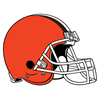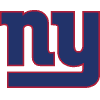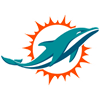DraftKings Make Playoffs Odds
-10000
0.01 to 1Buccaneers
93.7% implied probability

-5000
0.02 to 1Cardinals
91.5% implied probability

-5000
0.02 to 1Bills
91.5% implied probability

-3500
0.03 to 1Rams
91.4% implied probability

-2000
0.05 to 1Packers
89.6% implied probability

-2000
0.05 to 1Cowboys
89.6% implied probability

-1200
0.08 to 1Titans
88.7% implied probability

-650
0.15 to 1Ravens
82.7% implied probability

-500
0.2 to 1Chargers
78.9% implied probability

-200
0.5 to 1Bengals
63.4% implied probability

-200
0.5 to 1Chiefs
63.0% implied probability

-175
0.57 to 1Browns
59.4% implied probability

-150
0.67 to 1Saints
56.9% implied probability

-110
0.91 to 1Raiders
50.0% implied probability

+125
1.25 to 1Colts
42.6% implied probability

+150
1.5 to 1Vikings
37.9% implied probability

+250
2.5 to 149ers
26.9% implied probability

+275
2.75 to 1Falcons
25.0% implied probability

+300
3 to 1Seahawks
23.8% implied probability

+300
3 to 1Steelers
23.8% implied probability

+350
3.5 to 1Patriots
21.1% implied probability

+350
3.5 to 1Broncos
21.1% implied probability

+450
4.5 to 1Eagles
17.5% implied probability

+650
6.5 to 1Panthers
12.8% implied probability

+650
6.5 to 1Bears
12.8% implied probability

+1000
10 to 1Football Team
8.5% implied probability

+1800
18 to 1Giants
5.1% implied probability

+2800
28 to 1Dolphins
3.4% implied probability

+5000
50 to 1Jaguars
1.9% implied probability

+10000
100 to 1Jets
% implied probability

+10000
100 to 1Texans
% implied probability

+25000
250 to 1Lions
% implied probability